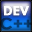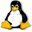Home Latest News Archive Tutorials C++ 2 3 4 Win32 Java OpenGL GLUT OpenGL ES 2 3 4 DirectX VB.NET HTML Photoshop 3dsmax Forums Projects Modelling Links About Us Search Recent Tutorials C / C++ Win32 OpenGL OpenGL ES GLUT## Pointers

Tutorials > C++ > Pointers

### What is a Pointer?

A pointer is a 4 byte address that points to a location in memory.

### Why are Pointers used?

Everytime you declare a variable, space is allocated on the stack. This space is limited and therefore you could run out of space on the stack when creating too many variables or variables of great size. Pointers allow you to reference data stored in memory which has a much greater capacity. The access speed is also much greater. You are therefore left with one 4 byte pointer on the stack which points to a large amount of data in memory.

### How are Pointers declared?

A pointer takes the form of :

<data type> *<variable_name>;

eg.

int *x;

will create a pointer that can address an integer in memory.

Contents of main.cpp :

```#include <iostream>
#include <stdlib.h>

using namespace std;

int main()
{
```

You usually need to allocate space in memory when wanting to point to new data. This will be covered in a later tutorial.
An easy way to create a string is to create a variable of type char * and assign a string to it. This will allocate a space in memory containing the string you requested. This is known as a C string. The string should not be edited however as the memory may be being used by more than one application.
Using code that is shown in comments will result in a runtime error.

```	// Different Character Pointers
//------------------------------

char *pointerToString = "Hello";
// pointerToString = 'r'; - RUNTIME ERROR
```

To prevent yourself from getting runtime errors created by the above problem, you can define all strings as being a const char *.
If you then try to use the code shown in comments, the compiler will give an error indicating that you cannot edit the string. This is much more useful than having your program crash at runtime, especially if the piece of code is not always executed.

```	const char *pointerToConstantString = "Hello";
// pointerToConstantString = 'r'; // COMPILE ERROR
```

If you want to create a pointer that can only ever point to one location, you can include the const keyword after the *. In our example, it will prevent you from reassigning a different string to the variable.

```	char * const constantPointerToString = "Hello";
// constantPointerToString = 't'; // RUNTIME ERROR
// constantPointerToString = "Hello2"; // COMPILE ERROR
```

This can be combined with our method of avoiding runtime errors as shown below.

```	const char * const constantPointerToConstantString = "Hello";
//constantPointerToConstantString = 'r'; // COMPILE ERROR
//constantPointerToConstantString = "Hello2"; // COMPILE ERROR
```

The cout object knows to output the data when a character pointer is given to it.

```	cout << pointerToConstantString << endl;
```

A few pointers have been defined to help us with the rest of the tutorial. The keyword NULL is used to indicate nothing. The px pointer therefore points to nothing.

```	// Pointer Declarations
//----------------------
int x = 0;
int *px = NULL;
char *str = "Hello World";
```

The following piece of code shows what happens when you output the size of a pointer. It should display both as being 4 bytes. It is important to note that when using the sizeof method on a char *, the length of the string is NOT outputted.

```	// Size of pointers
//----------------------
cout << "Size of pointer : "
<< sizeof(px) << endl;
cout << "Size of str : "
<< sizeof(str) << endl;
```

The next piece of code shows what happens when you output pointers. x outputs 0 as it is a normal integer that has been assigned the value of 0. px however, outputs a number of 0s. This is because it is an address (NULL address).

```	// Values
//--------
cout << "Value of x : "
<< x << endl;
cout << "Value of px : "
<< px << endl;
```

If you want to use the address of a variable, you need to place a & before the variable name.
&x and &px therefore output memory locations. I received the values 0012FEA4 and 0012FE98 but these will probably be different on your machine.
Notice that &px will give you the address of the pointer ie. where the pointer is located. It will not give you the address that it points to. If you want to use the address that it points to, you need to use px without the &.

```	// Addresses
//-----------
cout << "Address of x : "
<< &x << endl;
cout << "Address of px : "
<< &px << endl;
```

If you would like to receive that actual value that is being pointed to, you need to place the * (dereference) operator before the variable name. str is a pointer to the first character of a string. *str will therefore output the first character which is in this case 'H'.
As a pointer is just an integer address, arithmetic can be used on the address. An example would be to add 1 to the pointer. (str + 1) will therefore give you the address of the second character of the string. If you dereference this you will receive an 'e'. A proper explanation of arithmetic will be done in a separate tutorial.

```
// Information pointed to
//------------------------
cout << "Value pointed to by str : "
<< *str << endl;
cout << "Value pointed to by str+1 : "
<< *(str + 1) << endl;

system("pause");

return 0;
}```

You should now know what a pointer is and how it can be used. Pointers are used in almost every C / C++ application. We have not covered everything in connection with pointers but we will revisit them in future tutorials.

Please let me know of any comments you may have : Contact Me

 Source Files :< Tutorial 07 - Arrays Tutorial 09 - Input >